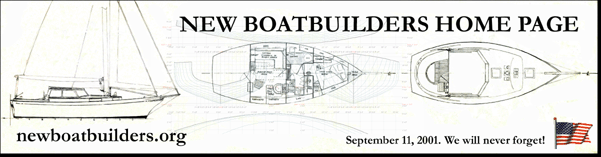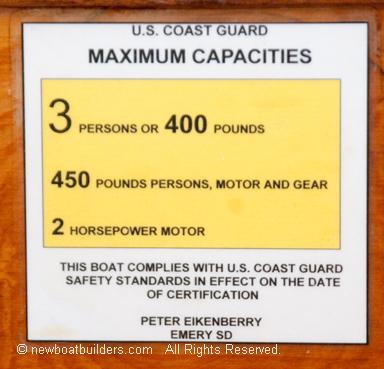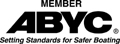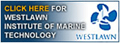Disclaimer:   I am not a spokesperson for the US Coast Guard or ABYC. For an official interpretation of regulations or standards you must contact the US Coast Guard or other organization referenced..   More.....

## Building the FL12  Page 2

Calculating Capacities and Making labels

One of the more important tasks a builder must perform for a small boat is determining weight and persons capacity, calculating how much flotation is needed and making a label for the boat. Also attaching a Hull Identification Number (HIN) is required.

For back yard boat builders in the US, who are building a boat for themselves, an HIN can be obtained from the state office that registers boats.  When registering the boat tell them it is a home built boat. They will have you fill out a form declaring that you built the boat, and assign an HIN.

In Canada this number is available from the Canadian Office of Boating Safety: Canadian Office of Boating Safety Regulations

Calculating Capacities and Flotation:

Many people consider calculating capacities and flotation as some mysterious scientific process beyond the capabilities of the average boat builder. This is definitely not true. Anyone with a basic education can do it. If you can add and subtract, multiply and divide, you can calculate boat capacities and flotation. For a boat like the FL 12 it is even simpler because the shapes are all squares, rectangles and triangles. You need to be able to find area and volume.

Area of any rectangle is the length times width.

Area of a square is one side times itself (or length times width but in this case the length equals the width).

The area of a triangle equals the base of the triangle (the bottom width) times the height, divided by two.

Volume involves one more dimension, the thickness or depth. So the volume of a box is the length of the box times the width times the depth.

The same applies to a triangular shapes but it depends on whether it is a three sided shape or a four sided shape.

Any boat like the FL12 that has a flat bottom, or even a vee bottom can be divided up into these shapes and the volume of each shape is found. Then the volumes are added up. The total volume times the weight of water (62.4 pounds per cubic foot) equals the displacement.

Displacement is the weight of the water that is moved out of the way when you make a hole in the water by putting a boat in it. Displacement divided by five is the maximum weight capacity. If you subtract the weight of the engine, battery, and a portable fuel tank, you get maximum persons capacity. That weight plus 32 and divided by 141 equals the number of person.

Of course the simple way to do this is put weight in the boat. Add weights until water is about to come in. The total weight is the displacement weight. Divide the total by five, and that gives you maximum weight capacity.

Another easy way to find persons capacity is to put weight on one side of the boat as far outboard as possible. Add weight until the boat heels to the point where water comes in. Divide that weight by 0.6 (or multiply by 1.6667) and that is the maximum persons capacity.

For the FL 12 I divided the boat into 10 equal sections. Each section forms a box. Then I measured the dimensions of each box to get the volume.

Starting at the bow. The bow section forms a triangular shaped box. Box 1 is 10” by 18 Â½” by 21 Â½”

10” = 0.8333 ft

18 Â½ “ = 1.54 ft

21 Â½ = 1.79 ft

the formula is 0.8333 X 1.54 / 2 = 0.642

then multiply 1.79 X 0.642 / 2 = 0.5746 = volume of Box 1

Box number two is a little more complicated because one side is large than the other side. That is the rear side is bigger than the front side.

The front side is 21 Â½ by 18 Â¼ by 11 Â½. It is not a rectangle, but since two sides are the same length we can treat it the same as a rectangle by using the average of the length of the two unequal sides.

21 + 11 Â½ = 32 Â½

32 Â½ / 2 = 16 Â¼ = 1.354 front area

the rear side = 28 Â¾ by 22 by 18 Â½

28Â¾ + 22 = 50 Â¾

50 Â¾ / 2 = 25 3/8 = 2.115 rear area

2.115 + 1.354 = 3.469

3.469 / 2 = 1.7345 Avg. area

1.7345 x 18 Â¼ = 1.756 X 1.54 = 2.671 volume of box 2

And so on.

I did it both ways to get a comparison between using Simpsons Method, (A method engineers use for calculating volumes of irregular shapes, derived from Calculus)and doing it the simple way by adding up the shapes of each section of the boat.  See Flotation: Calculation Methods  For the FL-12 the Simpson calculations look like this (I did this in an Excel Spreadsheet)

Look at the middle of the table where it is outlined in red. On the far right are the displacements calculated both ways and they are within 2 lb. of each other. This is not a significant difference and makes no difference in the capacities and amount of flotation.

By Calculating volume;  volume equals 2545 lb. = displacement weight

Maximum Weight Capacity = 2445 times 3/10 = 733.5 lb.

Maximum Persons weight = (733.5 - 25) x 0.90 = 637.5 lb.

Maximum Persons = 638 + 32 / 141 = 4.75 persons

Note:  These are calculations for a boat with 2 HP or less. The formulas for a boat greater than 2 HP or an inboard with level flotation are different but the procedure is the same. see Calculating Flotation Needs.

 Calculations for Areas of Stations Calculations for displacement weight Using Simpsons Method Alternate Method Station No. Half Area Simpsons Function Functions volume by blocks 0 0.3120 1 0.312 sta 1 1.0420 4 4.168 bow 0.2535 2 1.5860 2 3.172 0-1 1.3940 3 1.9900 4 7.960 1-2 2.6700 4 2.2730 2 4.546 2-3 3.5800 5 2.4060 4 9.624 3-4 4.3570 6 2.4450 2 4.890 4-5 4.7700 7 2.3550 4 9.420 5-6 4.8370 8 2.0060 2 4.012 6-7 4.7990 9 1.9570 4 7.828 7-8 4.5380 10 1.6875 1 1.688 8-9 4.1670 9-10 3.6400 Total Simpsons functions 57.620 10-T 1.7483 volume from simpson 38.413 vol of transom 0.375 stern piece 1.748 bow piece 0.254 total vol 40.79 Total Vol 40.75 Distance btwn Stations= 1 Vol Added Vol Total Vol Buoyancy Displ Simpsons 0.67 38.413 2.377 40.79 62.4 2545.30 Alternate Method 40.75 62.4 2543.04 Weights are in pounds Simpsons Blocks Boat weight 100 100 Max disp -boat weight 2445.30 2443.04 Max Weight Capacity 733.59 732.91 Max Persons Capacity 637.73 637.12 Max Persons 4.75 4.75 K for Ply HP = 2 Engine Weight = 25 lb Buoyancy = 60.4 Hull Weight = Wh = -0.81 Wh K cu Ft Flotation for Boat Wh X K / 60.4 100 -0.81 -1.34106 Engine Wt Flotation For Engine 25 0.413907 Persons Wt Flotation for Persons 637.73 2.639611 Total Flotation 1.712458 Actual installed flotation 2 ft X 8 ft X 0.167 ft = Aft Mid forward Total 0.75 1.2 0.722 2.672

Making a label.  You can create the label on any inkjet printer.  You first create it in Microsoft Word, or other word processor and then print it out. See what the labels look like at Labels. You can download a word doc that has a blank label here.

1. Replace the numbers with the correct capacities.
2. Save Document
3. Print
4. Cut out Label
5. Laminate in plastic so it is waterproof.
6. Glue or fasten to boat. It must be permanently affixed.

It should look like this: